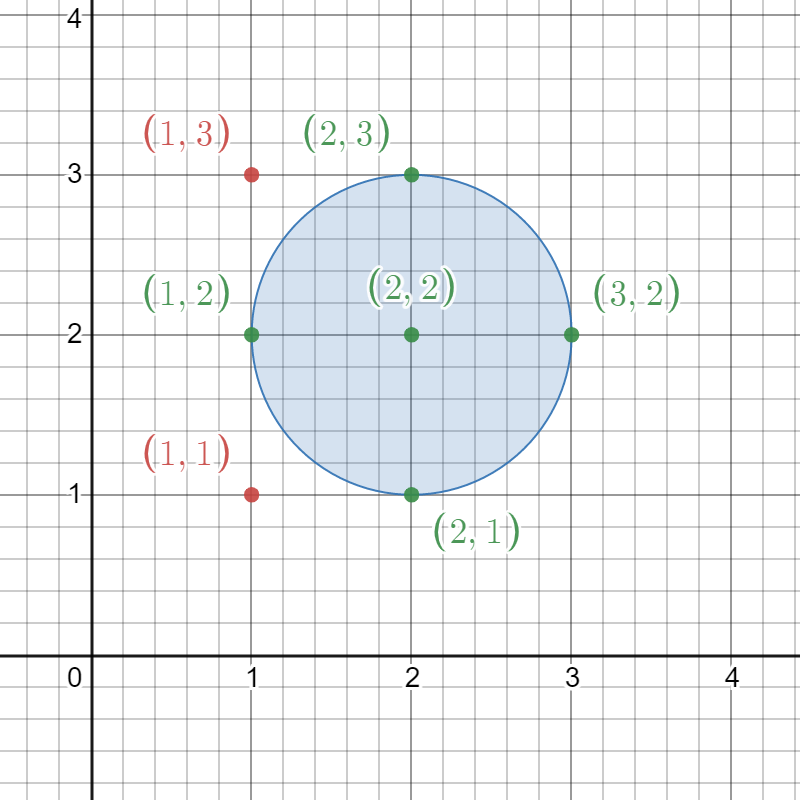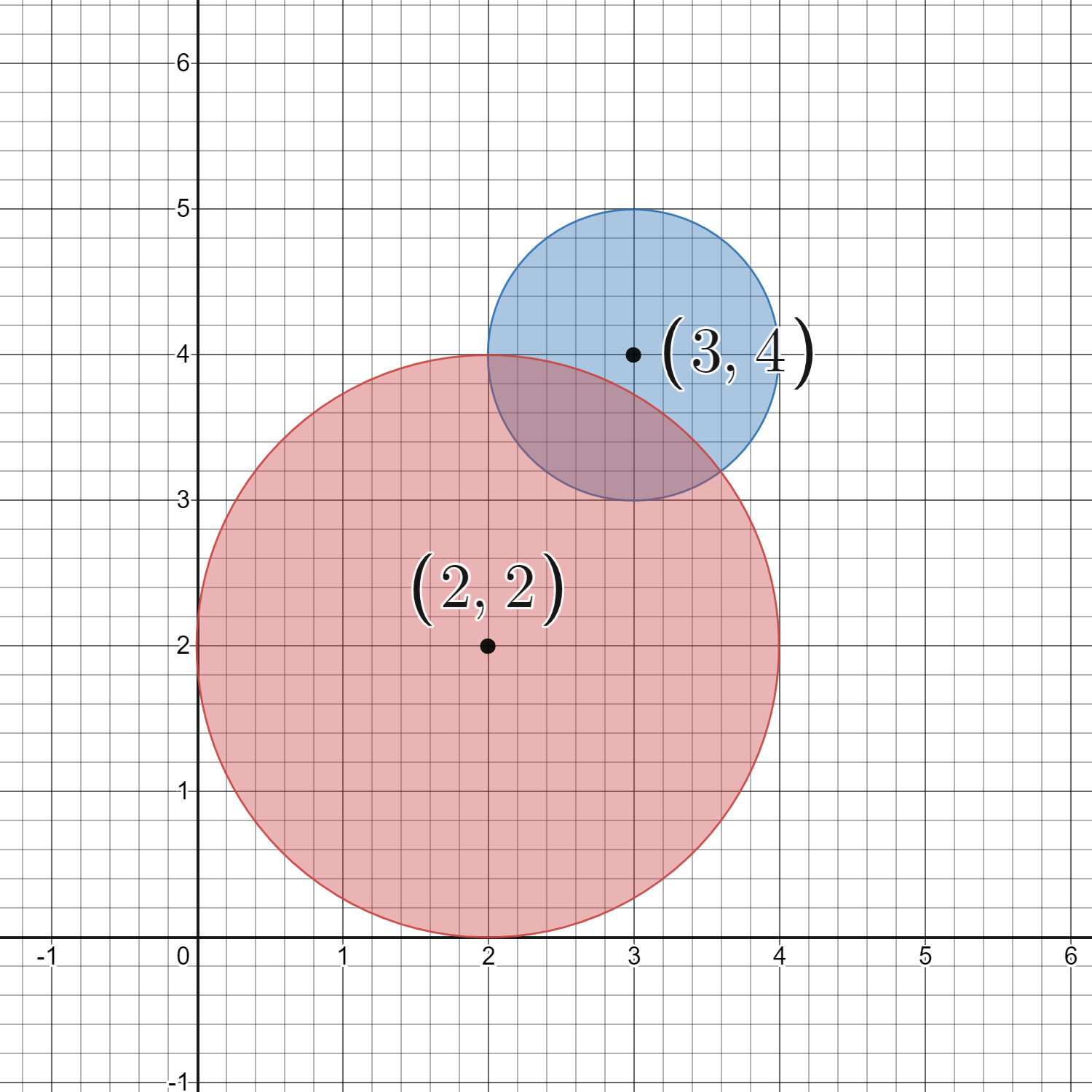2249. Count Lattice Points Inside a Circle
Medium
179
198

Given a 2D integer array `circles` where `circles[i] = [xi, yi, ri]` represents the center `(xi, yi)` and radius `ri` of the `ith` circle drawn on a grid, return the number of lattice points that are present inside at least one circle.

Note:

• A lattice point is a point with integer coordinates.
• Points that lie on the circumference of a circle are also considered to be inside it.

Example 1:```Input: circles = [[2,2,1]]
Output: 5
Explanation:
The figure above shows the given circle.
The lattice points present inside the circle are (1, 2), (2, 1), (2, 2), (2, 3), and (3, 2) and are shown in green.
Other points such as (1, 1) and (1, 3), which are shown in red, are not considered inside the circle.
Hence, the number of lattice points present inside at least one circle is 5.```

Example 2:```Input: circles = [[2,2,2],[3,4,1]]
Output: 16
Explanation:
The figure above shows the given circles.
There are exactly 16 lattice points which are present inside at least one circle.
Some of them are (0, 2), (2, 0), (2, 4), (3, 2), and (4, 4).
```

Constraints:

• `1 <= circles.length <= 200`
• `circles[i].length == 3`
• `1 <= xi, yi <= 100`
• `1 <= ri <= min(xi, yi)`
Accepted
18.3K
Submissions
36.2K
Acceptance Rate
50.6%

Seen this question in a real interview before?
1/4
Yes
No

Discussion (0)

Related Topics